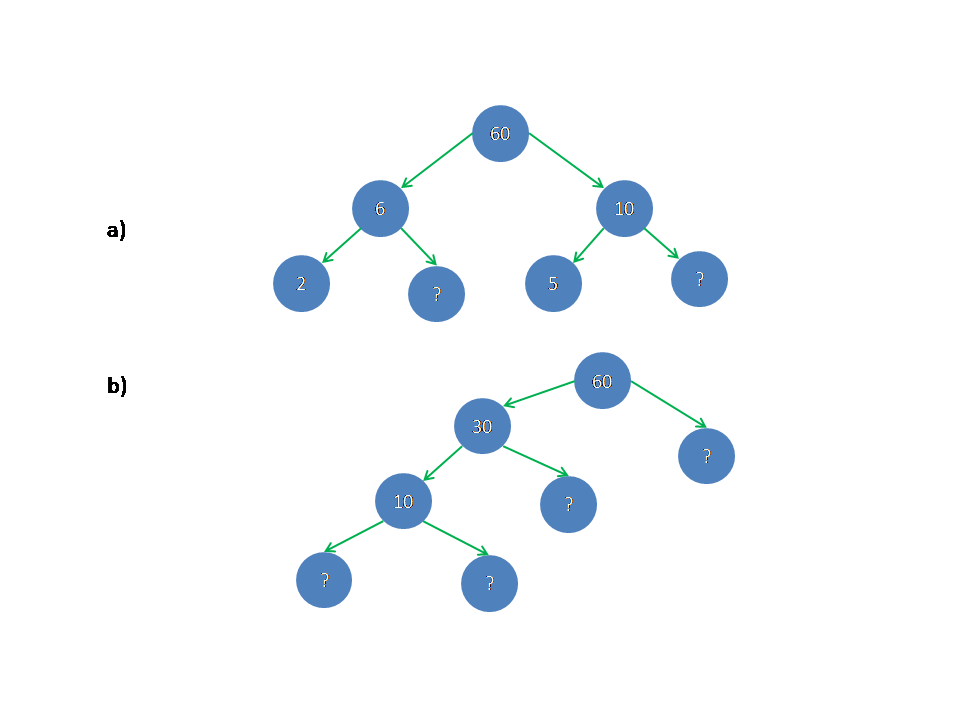# ncert cbse solutions## Ncert solutions for class 6th maths/chapter 3,Playing with Numbers solutions/factors

NCERT solutions for class 6th maths, playing with numbers, factors, chapter 3 Chapter 3 class 6 Playing with Numbers Maths NCERT solutions are given. First you study the textbook lesson very well. Observe and practice the example problems and solutions given in the textbook. 1. A factor of a number is an exact divisor of …

## NCERT solutions for class 6th maths chapter 2/  whole numbers solutions,problems with solutions of whole numbers

NCERT SOLUTIONS FOR CLASS 6TH CLASS MATHS CHAPTER 2, WHOLE NUMBERS SOLUTIONS Chapter 2 class 6 Maths NCERT Solutions for Whole Numbers are given. You should Study the textbook lesson Whole numbers very well. You should also observe the example problems and solutions given in the textbook. You can also see the solutions of some …

## NCERT solutions for class 6th maths chapter 1, Knowing our Numbers solutions

CHAPTER 1 NCERT SOLUTIONS FOR CLASS 6 MATHS CHAPTER 1, KNOWING OUR NUMBERS Solutions for Knowing our Numbers Chapter 1 class 6 Maths Ncert are given. You should study the textbook lesson Knowing our numbers very well. Practice the example Problems and solutions given in the textbook. SOLUTIONS OF KNOWING OUR NUMBERS FOR VI CLASS …